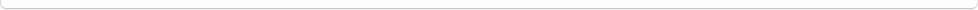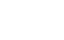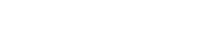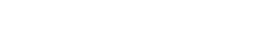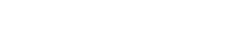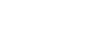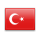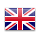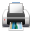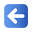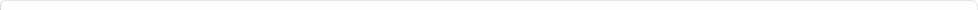Description of Individual Course Units
 Course Unit Code Course Unit Title Type of Course Unit Year of Study Semester Number of ECTS Credits 3351003104 DIGITAL ELECTRONICS Compulsory 2 3 3
Level of Course Unit
Short Cycle
Objectives of the Course
It aimed with this course that students will gain basic competence in basic logic circuits, simplifiying techniques of logic circuits, creating logic circuits, finding electrical equivalence, solving a problem and creating needed circuit and operating.
Name of Lecturer(s)
Öğr. Gör. Murat KARAKILIÇ
Learning Outcomes
 1 1-Students will create basic logic circuits. 2 2- Students will simplify logic circuits. 3 3-Students will solve logical problems, create logic circuit and operate.
Mode of Delivery
Evening Education
Prerequisites and co-requisities
None
Recommended Optional Programme Components
None
Course Contents
"A. NUMBER SYSTEMS 1. Numbers 2. Conversiton of Number Systems 3. Addition in Binary Number System 4. Subtraction in Binary Number System B. LOGICAL GATE CIRCUITS 1. Logical Gates 2. Logical Integre Types A. BOOLEAN MATEMATİĞİ 1. Boolean Operations 2. Boolean Laws 3. Boolean Mathematical Rules 4. De Morgan Theorem 5. Digital Circuit Design B. KARNOUGH MAP 1. Karnough Map according to Variables 2. Place Function on Karnough Map 3. Grouping on Karnough Map 4. Write Simplified Expression on Karnough Map 5. Karnough Map according to Spaces "
Weekly Detailed Course Contents
 Week Theoretical Practice Laboratory 1 Number Systems X 2 Number Systems X 3 Logical Door Circuits X 4 Logical Door Circuits X 5 Entegre devre aileleri ve teknik özellikleri X 6 Circuit drawing from logic functions Finding the logic function of a drawn circuit Circuit drawing from logic functions X 7 Transformations between logic circuits and electrical circuits X 8 Boolean Mathematics X 9 Boolean Mathematics X 10 Map of Karnaugh X 11 Map of Karnaugh X 12 To remove the logic function of a problem and simplify it X 13 Bir problemin zaman diyagramını oluşturmak X 14 To establish and operate a logic circuit of a problem X
Dijital Elektronik- Mustafa YAĞIMLI, Feyzi AKAR
Planned Learning Activities and Teaching Methods
Assessment Methods and Criteria
 Term (or Year) Learning Activities Quantity Weight Midterm Examination 1 100 SUM 100 End Of Term (or Year) Learning Activities Quantity Weight Final Examination 1 100 SUM 100 Term (or Year) Learning Activities 40 End Of Term (or Year) Learning Activities 60 SUM 100
Language of Instruction
Turkish
Work Placement(s)
None